# Python tkinter pack

Basics of Python Tkinter

Managing layout while using widgets by using Pack.

Tkinter pack layout manager with option fill side expand anchor to place widgets in different places

## Understanding Pack

Without any options all widgets will be placed vertically in a column. We can add side , fill and expand to manage the layout.

## side

 `side=LEFT` Placed at LEFT of Next element `side=RIGHT` Placed at RIGHT of Next element `side=BOTTOM` Placed at BOTTOM of next element `side=TOP` Placed at TOP of next element

### side=left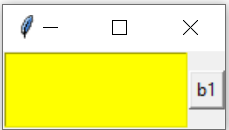``````import tkinter as tk
from tkinter import *
my_w=tk.Tk()
# Placed at LEFT of Next element b1
l1 = tk.Listbox(my_w,height=3,bg='yellow')
l1.pack(side=LEFT)

# Immaterail as no next element
b1=tk.Button(my_w,text='b1')
b1.pack(side=RIGHT)
my_w.mainloop()``````

### side=LEFT ( three elements )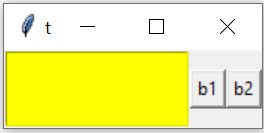``````# Placed at LEFT of next b1
l1 = tk.Listbox(my_w,height=3,bg='yellow')
l1.pack(side=LEFT)

# Placed at LEFT of Next element  b2
b1=tk.Button(my_w,text='b1')
b1.pack(side=LEFT)

b2=tk.Button(my_w,text='b2')
b2.pack(side=RIGHT) # Immaterial
my_w.mainloop()``````

### side=RIGHT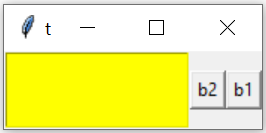``````l1 = tk.Listbox(my_w,height=3,bg='yellow')
l1.pack(side=LEFT)
# Next element will be placed RIGHT of this l1

b1=tk.Button(my_w,text='b1')
b1.pack(side=RIGHT)
# Next element ( b2 ) will be left of this b1

b2=tk.Button(my_w,text='b2')
b2.pack(side=RIGHT)
# Next element ( not there) so Immaterial
my_w.mainloop()``````

### side=BOTTOM``````# Next element will be placed RIGHT of this l1
l1 = tk.Listbox(my_w,height=3,bg='yellow')
l1.pack(side=LEFT)

b1=tk.Button(my_w,text='b1')
b1.pack(side=BOTTOM)
#Takes Next row relative to next element ( b2 )

b2=tk.Button(my_w,text='b2')
b2.pack(side=RIGHT) # Immaterial
#b2 will be above b1``````

## fill

 `fill=X` Expand horizontally to fill the available space `fill=Y` Expand vertically to fill the available space `fill=BOTH` Expand horizontally & vertically to fill the available space

### fill=X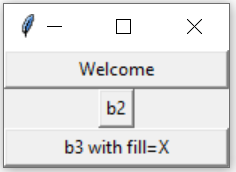``````import tkinter as tk
my_w=tk.Tk()
b1=tk.Button(my_w,text="Welcome",width=20)
b1.pack() # Occupies the assigned width

# in the absence of any side value for b1, b2 starts in next row
b2=tk.Button(my_w,text="b2")
b2.pack()    # Width is as minimum, no expansion

b3=tk.Button(my_w,text="b3 with fill=X")
b3.pack(fill=X) # fill all horizontal space
my_w.mainloop()``````

### fill=Y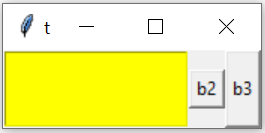``````l1 = tk.Listbox(my_w,height=3,bg='yellow')
l1.pack(side=LEFT) # Occupies the assigned width

# in the absence of any side value for b1, b2 starts in next row
b2=tk.Button(my_w,text="b2")
b2.pack(side=LEFT)    # Width is as minimum, no expansion

b3=tk.Button(my_w,text="b3")
b3.pack(side=LEFT,fill=Y) # fill all vertical space
my_w.mainloop()``````

## expand

Default value is False.
The expand option ( if True or 1 ) tells the manager to assign additional space to the widget box. If the parent widget is made larger than necessary to hold all packed widgets, any exceeding space will be distributed among all widgets that have the expand option set to a non-zero value.

### Difference between expand and fill

expand option ( if set to True ) tells to take any not assigned space .
The option fill asks the widget to take as much space as possible in given direction.

## Align at top left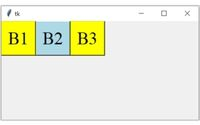Three side by side buttons are aligned at top left corner of the window
``````b1=tk.Button(my_w,bg='yellow',text='B1')
b1.pack(side='left',anchor='nw')
b2=tk.Button(my_w,bg='lightblue',text='B2')
b2.pack(side='left',anchor='nw')
b3=tk.Button(my_w,bg='yellow',text='B3')
b3.pack(side='left',anchor='nw')``````

## Align at top right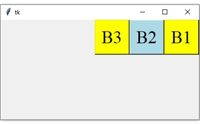``````b1=tk.Button(my_w,bg='yellow',text='B1')
b1.pack(side='right',anchor='ne')
b2=tk.Button(my_w,bg='lightblue',text='B2')
b2.pack(side='right',anchor='ne')
b3=tk.Button(my_w,bg='yellow',text='B3')
b3.pack(side='right',anchor='ne')``````

## Align at bottom right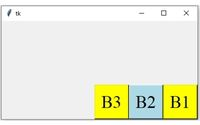``````b1=tk.Button(my_w,bg='yellow',text='B1')
b1.pack(side='right',anchor='se')
b2=tk.Button(my_w,bg='lightblue',text='B2')
b2.pack(side='right',anchor='se')
b3=tk.Button(my_w,bg='yellow',text='B3')
b3.pack(side='right',anchor='se')``````

## Align at bottom left``````b1=tk.Button(my_w,bg='yellow',text='B1',font=font1)
b1.pack(side='left',anchor='sw')
b2=tk.Button(my_w,bg='lightblue',text='B2',font=font1)
b2.pack(side='left',anchor='sw')
b3=tk.Button(my_w,bg='yellow',text='B3',font=font1)
b3.pack(side='left',anchor='sw')``````

## pack_forget() and destroy()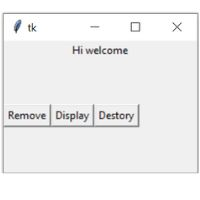By using pack_forget() we can remove the widget from layout. The widget is not destroyed so we can restore the same. Here is a code to display and hide the message on button click. On click of button b1 the code `command=lambda: l1.pack_forget()` removes the widget. We can restore it by using pack() `command=lambda: l1.pack()`.
We can delete the widget by using delete(). `command=lambda: l1.destroy()`
``````import tkinter as tk
from tkinter import *
my_w=tk.Tk()
my_w.geometry("220x150")
my_str = tk.StringVar(value='Hi welcome')
l1 = tk.Label(my_w,  textvariable=my_str, width=20 )
l1.pack(side=TOP)
b1=tk.Button(my_w,text='Remove',
command=lambda: l1.pack_forget() )
b1.pack(side=LEFT)

b2=tk.Button(my_w,text='Display',
command=lambda: l1.pack())
b2.pack(side=LEFT)

b3=tk.Button(my_w,text='Destory',
command=lambda: l1.destroy())
b3.pack(side=LEFT)

my_w.mainloop()``````

## pack_info()

Get all information as a dictionary about the widget.
``print(b1.pack_info())``
Output , option names with values as key value pairs of a dictionary.
``````{'in': , 'anchor': 'center', 'expand': 0, 'fill': 'none',

## pack_propagate(flag)

If the flag is set then it will resize the manager to hold all the widgets. This should be called from master widget as it controls the manager
``````import tkinter as tk
my_w=Tk()
l1 = tk.Listbox(my_w,height=3,bg='yellow')
l1.pack(side=LEFT) #

b1=tk.Button(my_w,text='RIGHT')
b1.pack(side=RIGHT) # Placed right of Listbox

my_w.pack_propagate(0)

my_w.mainloop()``````

## pack_slaves()

get a list of widgets
``print(my_w.slaves())``
Output
``[<tkinter.Listbox object .!listbox>, <tkinter.Button object .!button>]``

## pack options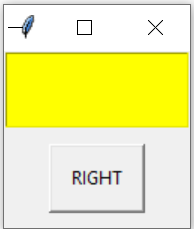We have seen above about fill and side. Other options are listed here. ( Check pack_info() above to get dictionary of options with values `print(b1.pack_info())` )

in : to pack widget inside
side : which side the widget is to be packed

Here is an example of using these values
``````import tkinter as tk
from tkinter import *
my_w=Tk()
l1 = tk.Listbox(my_w,height=3,bg='yellow')
l1.pack(side=TOP) #

b1=tk.Button(my_w,text='RIGHT')
b1.pack(side=RIGHT,anchor='center',expand=1,fill=Y,
my_w.mainloop()``````
The output of `print(b1.pack_info())` is here .
``````{'in': <tkinter.Tk object .>, 'anchor': 'center', 'expand': 1,

Subscribe to our YouTube Channel here

## Subscribe

* indicates required
Subscribe to plus2netplus2net.com